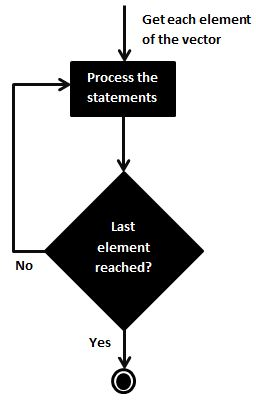# R - For Loop

A For loop is a repetition control structure that allows you to efficiently write a loop that needs to execute a specific number of times.

## Syntax

The basic syntax for creating a for loop statement in R is −

```for (value in vector) {
statements
}
```

## Flow DiagramR’s for loops are particularly flexible in that they are not limited to integers, or even numbers in the input. We can pass character vectors, logical vectors, lists or expressions.

## Example

```v <- LETTERS[1:4]
for ( i in v) {
print(i)
}
```

When the above code is compiled and executed, it produces the following result −

``` "A"
 "B"
 "C"
 "D"
```
r_loops.htm